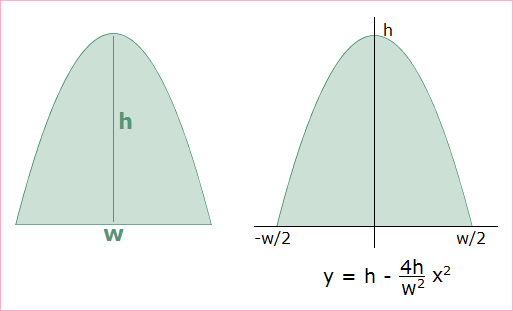# Arc Length and Area of Parabolic Segment

Parabolic Segment Calculator
Height
Width

A parabola is a curve in the family of conic sections. There are several ways to describe the formation of a parabolic arc in terms of algebraic equations, plane geometry, and solid geometry.

If you slice a cone along a plane parallel to the side of the cone, the resulting cross-sectional shape is a parabola. If you consider the set of points in the xy-plane that are equidistant from a given line and point outside that line (the directrix and focus respectively), the set of points is a parabola. In algebra, the standard equation of a parabola is y = f(x) = ax² + bx + c.

A symmetric parabolic segment has endpoints that are equidistant from the vertex. If you know the height of the arc and the width between the two endpoints, you can compute the arc length and area of the segment.If you align the segment's axis of symmetry with the y-axis, you can write the equation of the parabola as

y = h - (4h/w²)x² on the interval [-w/2, w/2],

which allows you to set up and compute integrals for the area and arc length.

### Area of the Segment

Evaluate the integral ∫[h - (4h/w²)x²] dx over the interval [-w/2, w/2] to find the area bounded by the arc and the x-axis. The result is

Area = (2/3)hw.

Notice that this is larger than the area of a triangle with height h and width w, but less than the area of a rectangle with dimensions h and w. (The triangular and rectangular area formulas are (1/2)hw and hw, respectively.)

### Arc Length of the Segment

Evaluate the integral ∫√1 + (y')² dx over the interval [-w/2, w/2]. The exact integral is

∫√1 + βx² dx

where β = 64h²/w4. You can use either a table of integrals or a graphing calculator to find the length. For arbitrary values of h and w, the formula for parabolic arc length is

Arc Length = 0.5√16h²+w² + [w²/(8h)][Ln(4h + √16h²+w²) - Ln(w)].